# 论文引入

Batch Normalization (BN)在Inception v2网络中被提出，它的设计之初是为了解决Internal Covariate Shift问题，也就是在训练过程中，隐层的输入分布老是变来变去。因为深层神经网络在做非线性变换前的激活输入值随着网络深度加深或者在训练过程中，其分布逐渐发生偏移或者变动，这导致了训练收敛慢，也就是数据整体分布逐渐往非线性函数的取值区间的上下限两端靠近。所以这导致反向传播时低层神经网络的梯度消失，这是训练深层神经网络收敛越来越慢的本质原因。

• 可以根据输入图像和语义图指导合成语义信息下的图像。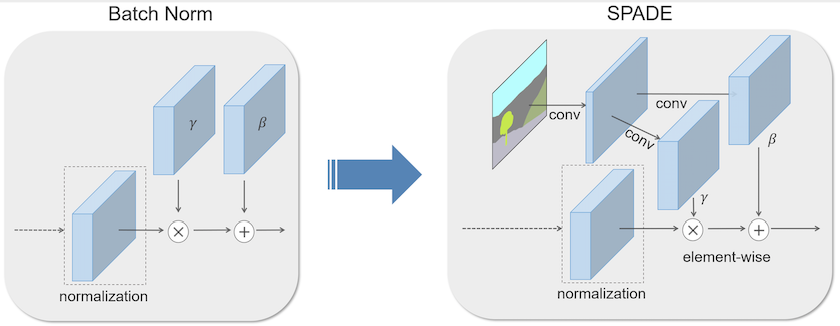$\gamma_{c,y,x}^i(m) \frac{h_{n,c,y,x}^i-\mu_c^i}{\delta_c^i}+\beta_{c,y,x}^i(m)$

$\mu_c^i = \frac{1}{NH^iW^i}\sum_{n,y,x}h_{n,c,y,x}^i$ $\delta_c^i = \sqrt{\frac{1}{NH^iW^i}\sum_{n,y,x}(h_{n,c,y,x}^i)^2-(\mu_c^i)^2}$

$\gamma_{c,y,x}^i(m)$和$\beta_{c,y,x}^i(m)$是Spatially-Adaptive Normalization学习参数，它们是通过语义分割图$m$经过卷积层得到的参数，此时的$\gamma_{c,y,x}^i(m)$和$\beta_{c,y,x}^i(m)$是包含语义分割图的语义信息的，同时也是语义分割图的feature map表示，这样学习到的参数将保留语义分割图的充分的语义信息，也实现了输入的归一化处理。

# 语义图像的生成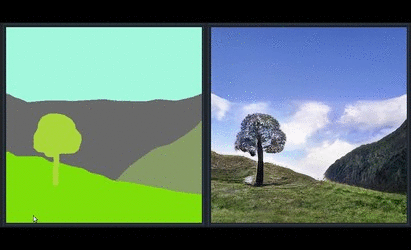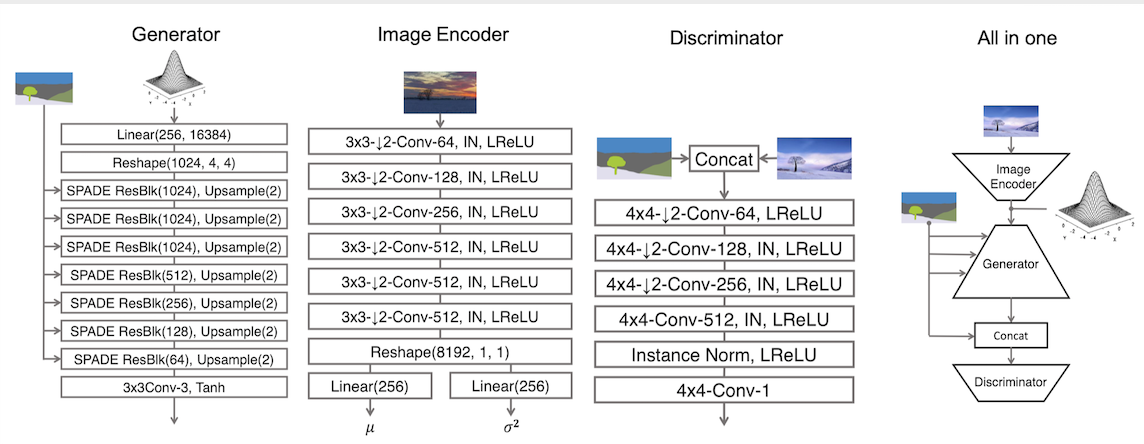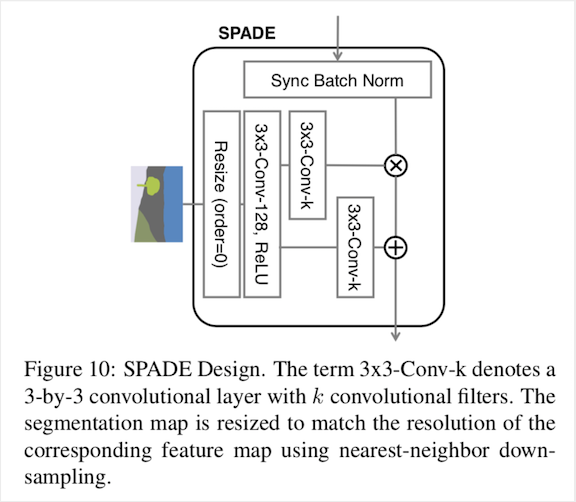def spade(segmap, x_init, channels, use_bias=True, sn=False, scope='spade'):
with tf.variable_scope(scope):
x = param_free_norm(x_init) # 进行均值，标准差计算，(x - x_mean) / x_std

_, x_h, x_w, _ = x_init.get_shape().as_list()
_, segmap_h, segmap_w, _ = segmap.get_shape().as_list()

factor_h = segmap_h // x_h  # 确定下采样的比例
factor_w = segmap_w // x_w

segmap_down = down_sample(segmap, factor_h, factor_w) # 最近邻下采样

# 第一步卷积处理
segmap_down = conv(segmap_down, channels=128, kernel=5, stride=1,
segmap_down = relu(segmap_down)

# 两个卷积得到segmap_gamma和segmap_beta
segmap_gamma = conv(segmap_down, channels=channels, kernel=5, stride=1,
segmap_beta = conv(segmap_down, channels=channels, kernel=5, stride=1,

# 引入残差
x = x * (1 + segmap_gamma) + segmap_beta

return x

def param_free_norm(x, epsilon=1e-5):
x_mean, x_var = tf.nn.moments(x, axes=[1, 2], keep_dims=True)
x_std = tf.sqrt(x_var + epsilon)

return (x - x_mean) / x_std

def down_sample(x, scale_factor_h, scale_factor_w):
_, h, w, _ = x.get_shape().as_list()
new_size = [h // scale_factor_h, w // scale_factor_w]

return tf.image.resize_nearest_neighbor(x, size=new_size)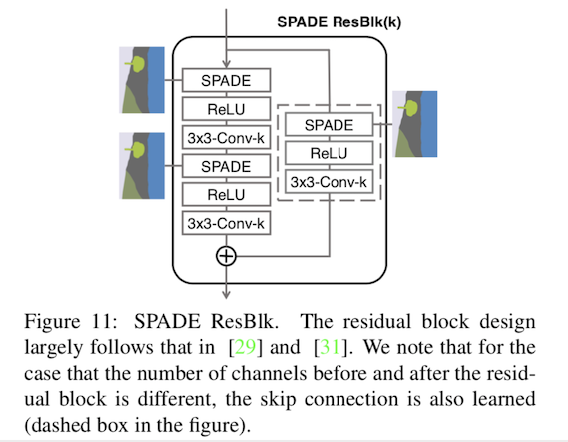def spade_resblock(segmap, x_init, channels, use_bias=True, sn=False, scope='spade_resblock'):
channel_in = x_init.get_shape().as_list()[-1]
channel_middle = min(channel_in, channels) # 由于是生成阶段，channel是逐渐变小的

with tf.variable_scope(scope):
x = lrelu(x, 0.2)
x = conv(x, channels=channel_middle, kernel=3, stride=1,

x = spade(segmap, x, channels=channel_middle, use_bias=use_bias,
x = lrelu(x, 0.2)
x = conv(x, channels=channels, kernel=3, stride=1, pad=1,
use_bias=use_bias, sn=sn, scope='conv_2')

if channel_in != channels:
x_init = spade(segmap, x_init, channels=channel_in, use_bias=use_bias,
x_init = conv(x_init, channels=channels, kernel=1, stride=1,
use_bias=False, sn=sn, scope='conv_shortcut')

return x + x_init



# 实验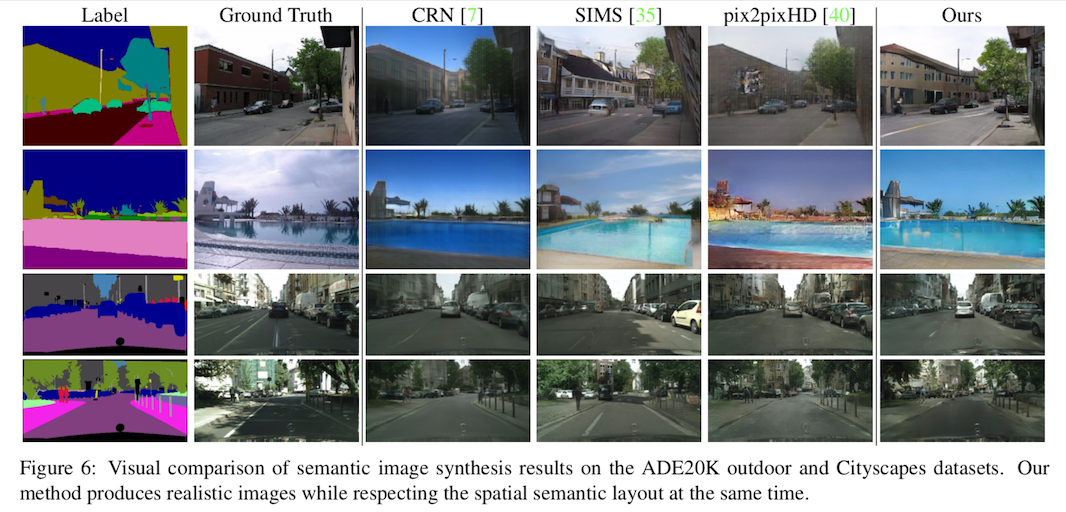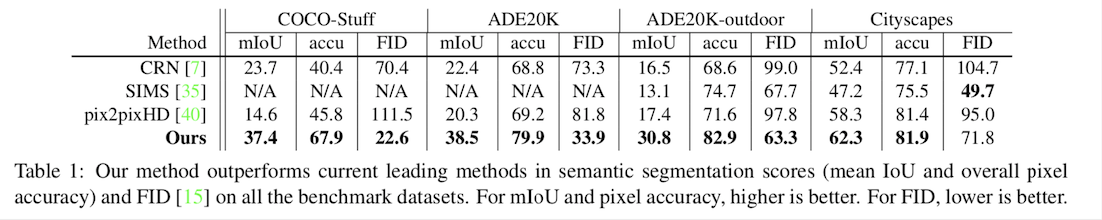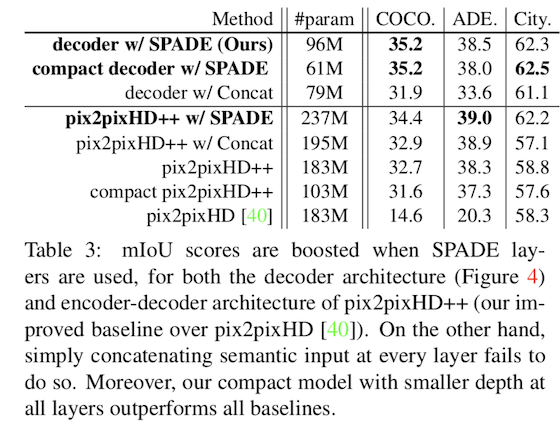# 参考文献

 S. Ioffe and C. Szegedy. Batch normalization: Accelerating deep network training by reducing internal covariate shift. In International Conference on Machine Learning (ICML), 2015.

 D. Ulyanov, A. Vedaldi, and V. Lempitsky. Instance nor- malization: The missing ingredient for fast stylization. arxiv 2016. arXiv preprint arXiv:1607.08022, 2016.

 V. Dumoulin, J. Shlens, and M. Kudlur. A learned repre- sentation for artistic style. In International Conference on Learning Representations (ICLR), 2016.

 Kingma D P, Welling M. Auto-encoding variational bayes[J]. arXiv preprint arXiv:1312.6114, 2013.

 Goodfellow I, Pouget-Abadie J, Mirza M, et al. Generative adversarial nets[C]//Advances in neural information processing systems. 2014: 2672-2680.

 T. Miyato, T. Kataoka, M. Koyama, and Y. Yoshida. Spec-tral normalization for generative adversarial networks. In In-ternational Conference on Learning Representations (ICLR), 2018.